• 若函数u=ϕ(t)、v=ψ(t)u=ϕ(t)、v=ψ(t)u = \phi(t)、v = \psi(t)都在点ttt可导，函数z=f(u,v)z=f(u,v)z=f(u,v)在对应点(u,v)(u,v)(u,v)具有连续偏导数，那么复合函数z=f[ϕ(t),ψ(t)]z=f[ϕ(t),ψ(t)]z=f[\phi(t),...
1、一元函数与多元函数复合的情形  若函数

u=ϕ(t)、v=ψ(t)

u

=

ϕ

(

t

)

、

v

=

ψ

(

t

)

$u = \phi(t)、v = \psi(t)$都在点

t

t

$t$可导，函数z=f(u,v)$z=f\left(u,v\right)$$z=f(u,v)$在对应点

(u,v)

(

u

,

v

)

$(u,v)$具有连续偏导数，那么复合函数

z=f[ϕ(t),ψ(t)]

z

=

f

[

ϕ

(

t

)

,

ψ

(

t

)

]

$z=f[\phi(t),\psi(t)]$在点

t

t

$t$可导，则对应z=f(u,v),{u=ϕ(t)v=ψ(t)$z=f\left(u,v\right),\left\{\begin{array}{l}u=\varphi \left(t\right)\\ v=\psi \left(t\right)\end{array}$z=f(u,v),
\begin{cases}
u = \phi(t)\\
v = \psi(t)
\end{cases}有

dzdt=∂z∂ududt+∂z∂vdvdt

d

z

d

t

=

∂

z

∂

u

d

u

d

t

+

∂

z

∂

v

d

v

d

t

\frac{dz}{dt} = \frac{\partial z}{\partial u}\frac{du}{dt} +\frac{\partial z}{\partial v}\frac{dv}{dt}

2、多元函数与多元函数复合的情形  若函数

u=ϕ(x,y)、v=ψ(x,y)

u

=

ϕ

(

x

,

y

)

、

v

=

ψ

(

x

,

y

)

$u = \phi(x,y)、v = \psi(x,y)$都在点

(x,y)

(

x

,

y

)

$(x,y)$具有对

x、y

x

、

y

$x、y$的偏导数，函数

z=f(u,v)

z

=

f

(

u

,

v

)

$z=f(u,v)$在对应点

(u,v)

(

u

,

v

)

$(u,v)$具有连续偏导数，那么复合函数

z=f[ϕ(x,y),ψ(x,y)]

z

=

f

[

ϕ

(

x

,

y

)

,

ψ

(

x

,

y

)

]

$z=f[\phi(x,y),\psi(x,y)]$在点

(x,y)

(

x

,

y

)

$(x,y)$的两个偏导数都存在，则对应

z=f(u,v),{u=ϕ(x,y)v=ψ(x,y)

z

=

f

(

u

,

v

)

,

{

u

=

ϕ

(

x

,

y

)

v

=

ψ

(

x

,

y

)

z=f(u,v),
\begin{cases}
u = \phi(x,y)\\
v = \psi(x,y)
\end{cases}有

∂z∂x=∂z∂u∂u∂x+∂z∂v∂v∂x

∂

z

∂

x

=

∂

z

∂

u

∂

u

∂

x

+

∂

z

∂

v

∂

v

∂

x

\frac{\partial z}{\partial x} = \frac{\partial z}{\partial u}\frac{\partial u}{\partial x} +\frac{\partial z}{\partial v}\frac{\partial v}{\partial x}

∂z∂y=∂z∂u∂u∂y+∂z∂v∂v∂y

∂

z

∂

y

=

∂

z

∂

u

∂

u

∂

y

+

∂

z

∂

v

∂

v

∂

y

\frac{\partial z}{\partial y} = \frac{\partial z}{\partial u}\frac{\partial u}{\partial y} +\frac{\partial z}{\partial v}\frac{\partial v}{\partial y}

展开全文高等数学
•文档
• 多元复合函数求导法则 注：复合函数为向量值函数。
注：复合函数为向量值函数。
链式求导法则
若：

m,p,n∈N,m,p,n≥1,
$m, p, n \in \mathbb N, m, p, n \ge 1,$

fm×1=⎛⎝⎜⎜f1⋮fm⎞⎠⎟⎟:Rp→Rm,gp×1=⎛⎝⎜⎜g1⋮gp⎞⎠⎟⎟:Rn→Rp,
$\mathbf{f}_{m \times 1} = \begin{pmatrix} f_1\\ \vdots\\ f_m \end{pmatrix} : {\mathbb R}^p \rightarrow {\mathbb R}^m, \mathbf{g}_{p \times 1} = \begin{pmatrix} g_1\\ \vdots\\ g_p \end{pmatrix} : {\mathbb R}^n \rightarrow {\mathbb R}^p,$

zm×1=f(yp×1),yp×1=g(xn×1),
$\mathbf{z}_{m \times 1} = \mathbf{f}(\mathbf{y}_{p \times 1}), \mathbf{y}_{p \times 1} = \mathbf{g}(\mathbf{x}_{n \times 1}),$

dz=f′(y)dy,dydx=(∂yi∂xj)p×n,
$\operatorname{d} \mathbf{z} = f'(\mathbf{y}) \operatorname{d} \mathbf{y}, \frac {\operatorname{d} \mathbf{y}}{\operatorname{d} \mathbf{x}} = (\frac {\partial y_i}{\partial x_j})_{p \times n},$
则：

dzdx=f′(g(x))g′(x)
$\frac {\operatorname{d} \mathbf{z}}{\operatorname{d} \mathbf{x}} = f'(g(\mathbf{x}))g'(\mathbf{x})$
证明：

∀i∈N,1≤i≤m,
$\forall i \in \mathbb N, 1 \le i \le m,$

Δzi=∑pk=1∂zi∂ykΔyk+∑pk=1Δyk2−−−−−−−−−√⋅αi(y,Δy)
$\Delta z_i = \sum _{k = 1}^ {p} \frac {\partial z_i}{\partial y_k}\Delta y_k + \sqrt {\sum _{k = 1}^ {p} {\Delta y_k}^2} \cdot \alpha _i(\mathbf{y}, \Delta \mathbf{y})$  其中

Δy=0
$\Delta \mathbf{y} = 0$ 时

αi(y,Δy)=0,
$\alpha _i(\mathbf{y}, \Delta \mathbf{y}) = 0,$ 且

limΔy→0αi(y,Δy)=0
$\lim _{\Delta \mathbf{y} \rightarrow 0} \alpha _i(\mathbf{y}, \Delta \mathbf{y}) = 0$  则：

∀j∈N,1≤j≤n,
$\forall j \in \mathbb N, 1 \le j \le n,$

ΔziΔxj=∑pk=1∂zi∂ykΔykΔxj+|Δxj|Δxj⋅∑pk=1(ΔykΔxj)2−−−−−−−−−−√⋅αi(y,Δy)
$\frac { \Delta z_i }{ \Delta x_j } = \sum _{k = 1}^ {p} \frac {\partial z_i}{\partial y_k} \frac { \Delta y_k }{ \Delta x_j } + \frac { | \Delta x_j | }{ \Delta x_j } \cdot \sqrt {\sum _{k = 1}^ {p} {(\frac { \Delta y_k }{ \Delta x_j })}^2} \cdot \alpha _i(\mathbf{y}, \Delta \mathbf{y})$  易知：

limΔxj→0Δy=0,
$\lim _{\Delta x_j \rightarrow 0} \Delta \mathbf{y} = 0,$ 则

limΔxj→0αi(y,Δy)=0
$\lim _{\Delta x_j \rightarrow 0} \alpha _i(\mathbf{y}, \Delta \mathbf{y}) = 0$  于是：

∂zi∂xj=limΔxj→0ΔziΔxj=∑pk=1∂zi∂yk∂yk∂xj
$\frac { \partial z_i }{ \partial x_j } = \lim _{\Delta x_j \rightarrow 0} \frac { \Delta z_i }{ \Delta x_j } = \sum _{k = 1}^ {p} \frac {\partial z_i}{\partial y_k} \frac { \partial y_k }{ \partial x_j }$  即

(dzdx)ij=∑pk=1(f′(g(x)))ik(g′(x))kj
$(\frac {\operatorname{d} \mathbf{z}}{\operatorname{d} \mathbf{x}})_{ij} = \sum _{k = 1}^{p} (f'(g(\mathbf{x})))_{ik} (g'(\mathbf{x}))_{kj}$
一阶全微分的形式不变性

dz=f′(g(x))g′(x)dx=f′(y)dy
$\operatorname{d} \mathbf{z} = f'(g(\mathbf{x}))g'(\mathbf{x}) \operatorname{d} \mathbf{x} = f'(\mathbf{y}) \operatorname{d} \mathbf{y}$
展开全文• 本篇内容为多元复合函数求导法则，内容其实难度还是不大的，但是作者看的时候有点费力，可能是因为我脑子慢吧，这篇写的时候我会尽可能的写的好理解一点，不管是我以后重新看还是大家拿去看都能省点心。...
本篇内容为多元复合函数的求导法则，内容其实难度还是不大的，但是作者看的时候有点费力，可能是因为我脑子慢吧，这篇写的时候我会尽可能的写的好理解一点，不管是我以后重新看还是大家拿去看都能省点心。
emmm想了下这一篇就不做关于一元函数求导的回顾了，一元函数求导显函数、隐函数、复合函数、参数方程函数，要回顾也不是一两句能说清楚的，推荐去看以前的相关内容，在第二章。
多元函数求导的内容也分为显函数、复合函数和隐函数，本篇中重点掌握后两者，因为显函数实在是没啥好说的。
显函数
给个例题就过了 例1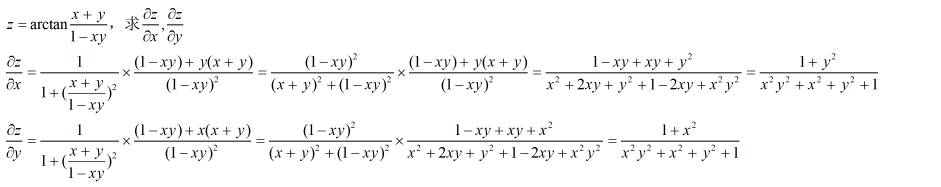例2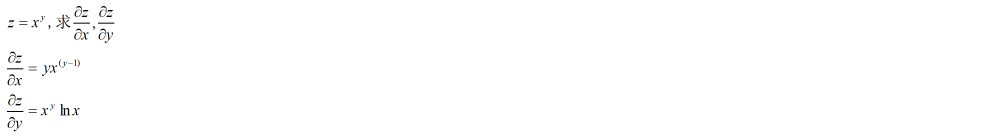多元显函数求偏导还是看求导的功力，没什么好说的，接下来是第二部分——多元复合函数求偏导。
多元复合函数求偏导
多元复合函数求偏导是有几种情形的，熟练以后根本不用分情形，但是刚开始接触，所以还是分一下。 情形一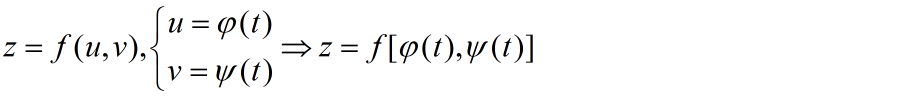这里有一个定理，这定理其实没啥用，不用记，会做题就行。 emmm还是给一下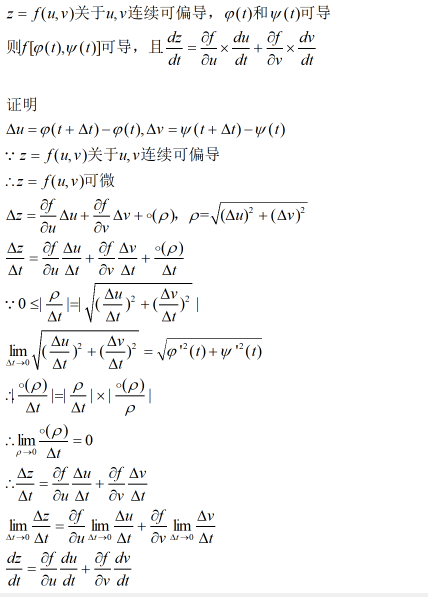注解1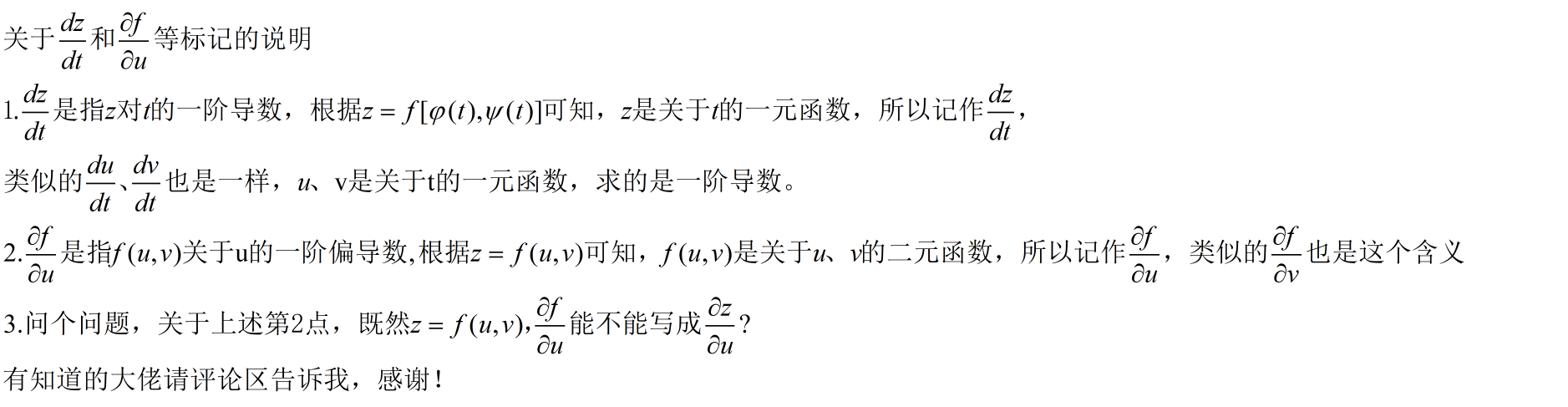注解2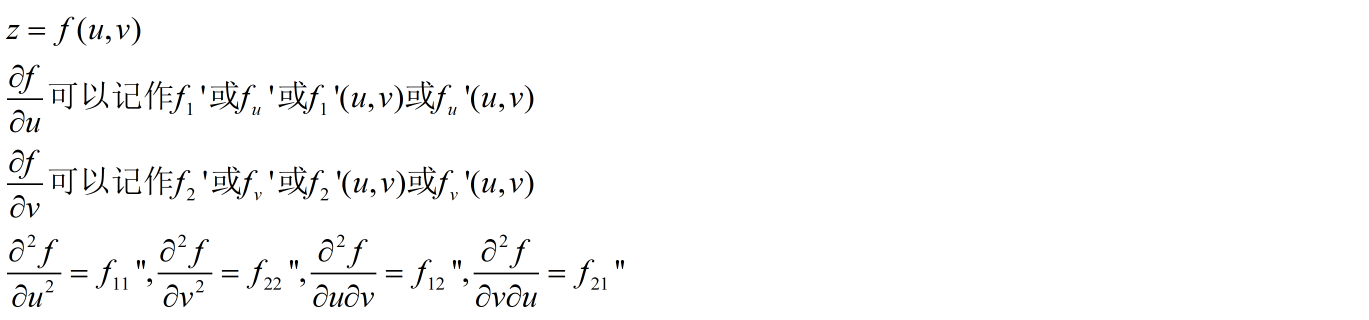例题
例2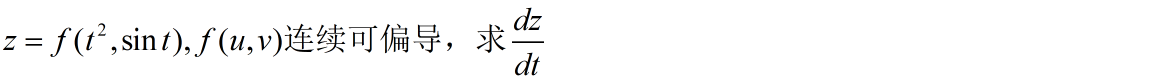画个图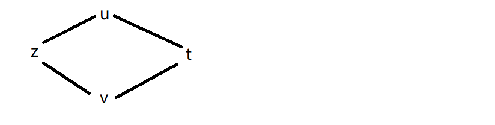z到t有两个路径，分别是z——u——t和z——v——t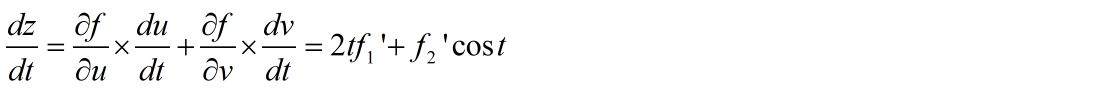情形二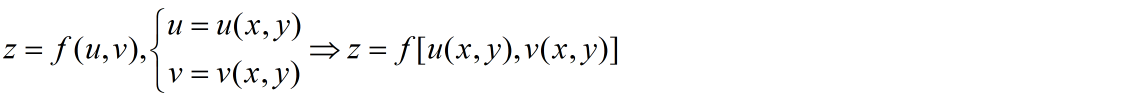定理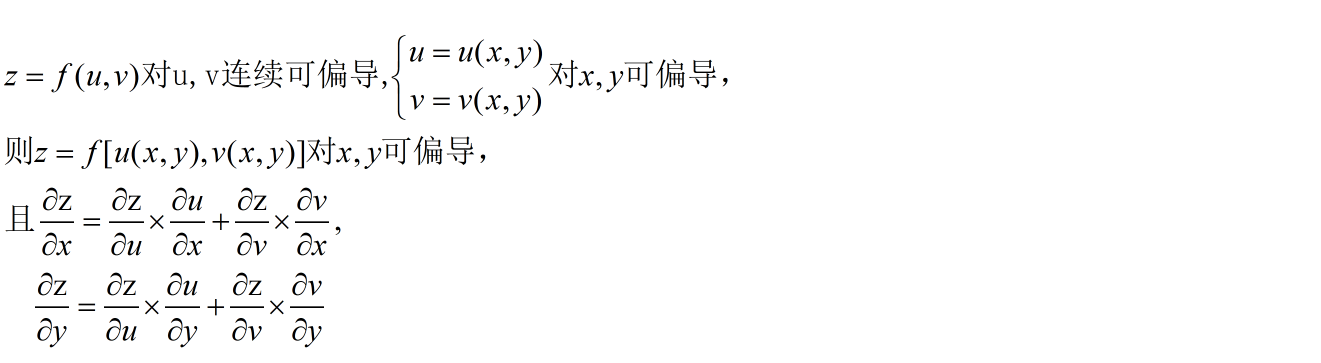给个图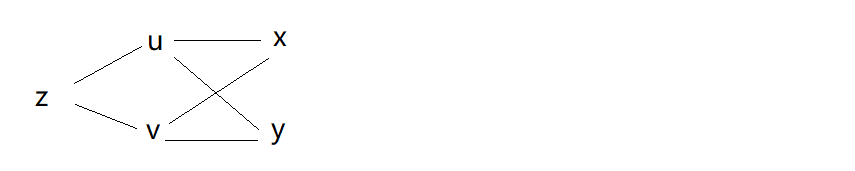例题
例3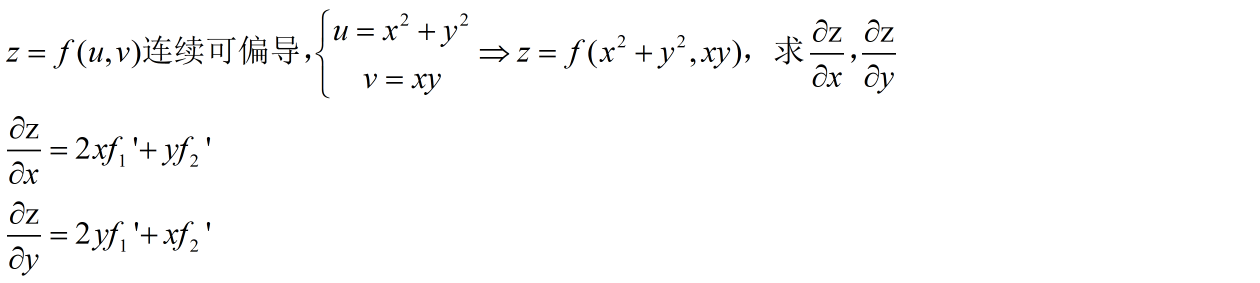例4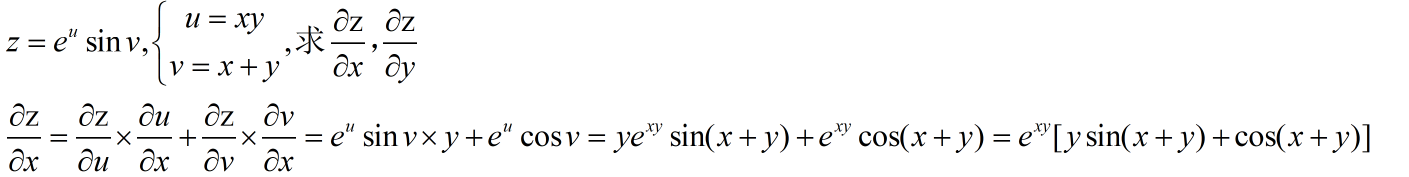例5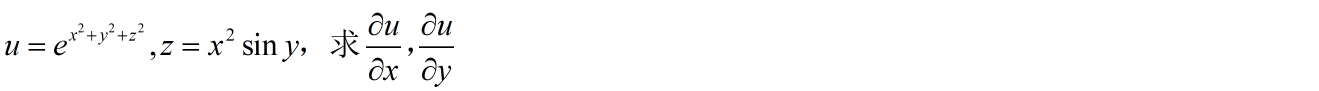画个图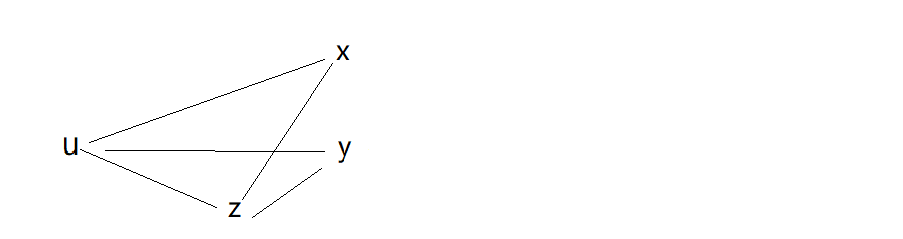例6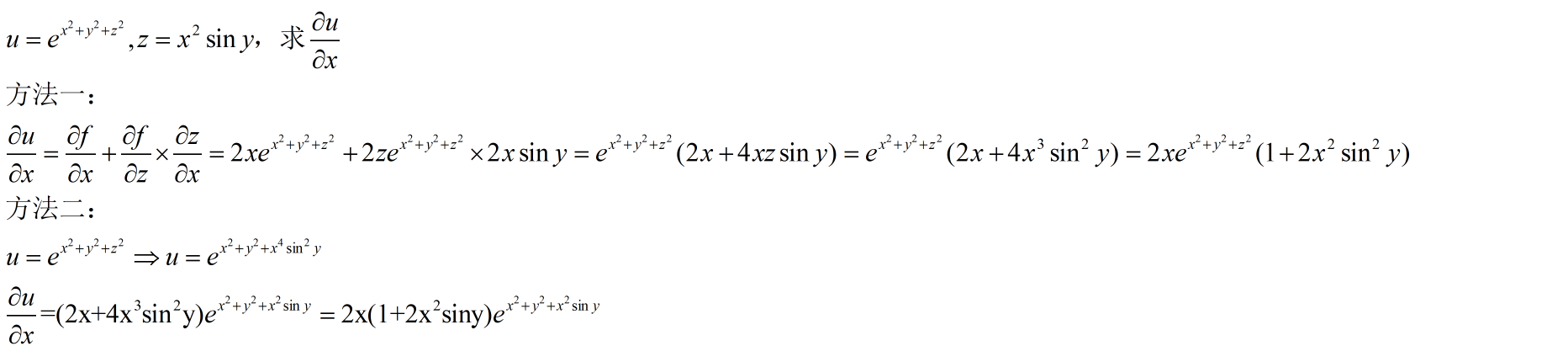例7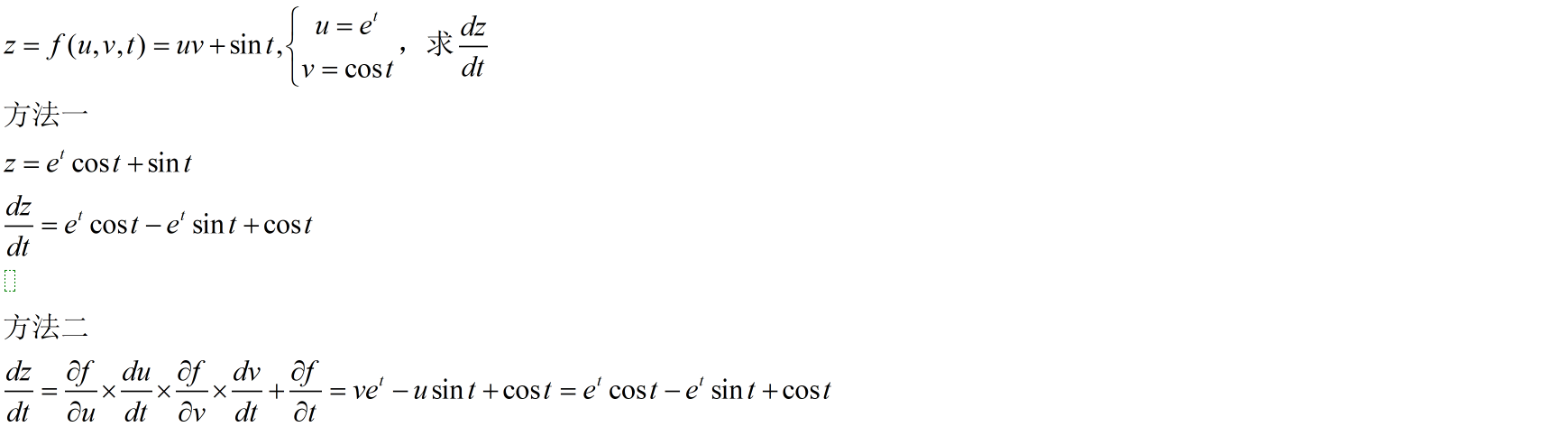例7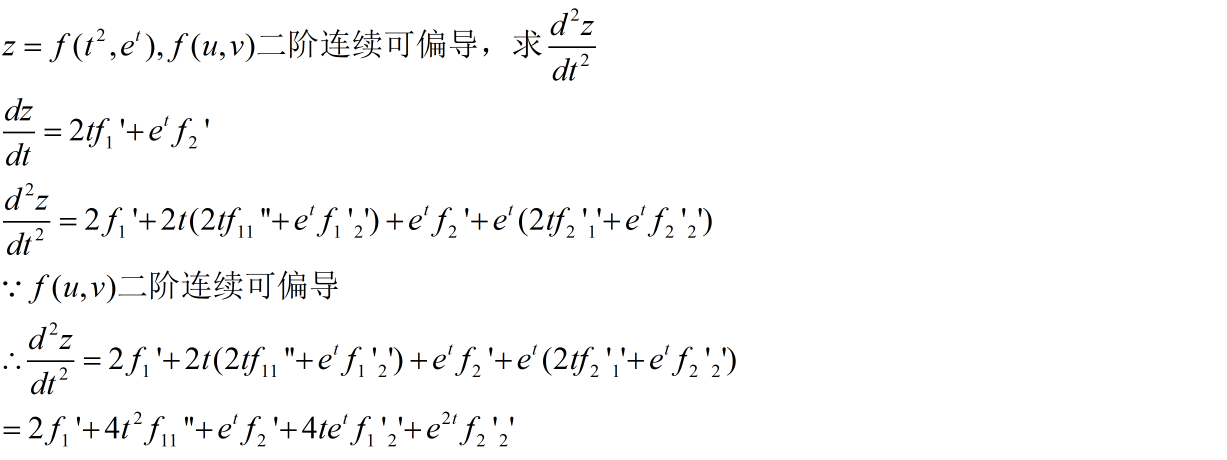例8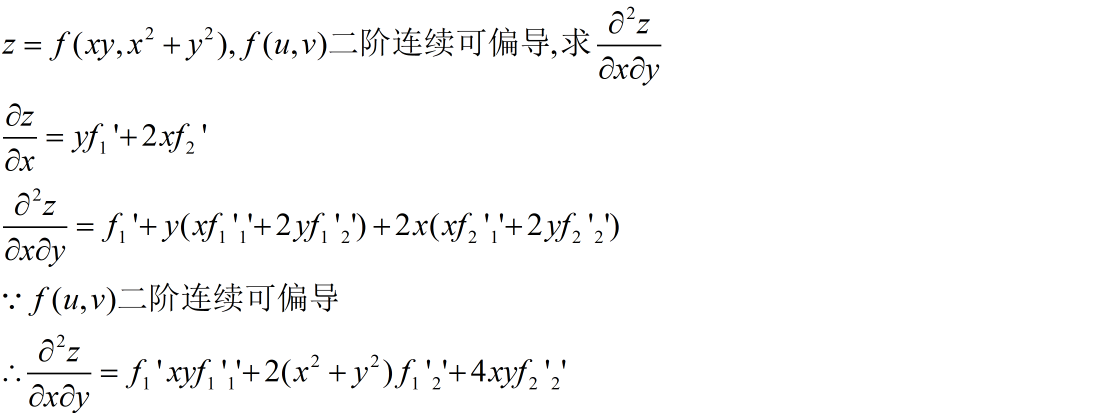总结
本篇内容注重练习，重点搞明白复合函数偏导的层次关系。本篇完。
展开全文• 多元复合函数求导法则


§第七章第四节多元复合函数的求导法则
$\color{blue}{\S 第七章 第四节 多元复合函数的求导法则}$

一元复合函数y=f(u),u=φ(x)求导法则dydx=dydu⋅dudx微分法则dy=f′(u)du=f′(u)φ′(x)dx
$一元复合函数 y = f(u), u = \varphi(x) \\ 求导法则\quad \dfrac{dy}{dx} = \dfrac{dy}{du} \cdot \dfrac{du}{dx} \\ 微分法则\quad dy = f^{\prime}(u)du = f^{\prime}(u)\varphi^{\prime}(x)dx$
熟练掌握多元复合函数求导的链式法则

定理.若函数u=φ(t),v=ψ(t)在点t可导,z=f(u,v)在点(u,v)处偏导连续,则复合函数z=f(φ(t),ψ(t))在点t可导,且有链式法则dzdt=∂z∂u⋅dudt+∂z∂v⋅dvdt
$定理.若函数u = \varphi(t),v = \psi(t)在点t可导, z=f(u, v)\\ 在点(u, v)处偏导连续,则复合函数z = f(\varphi(t), \psi(t))\\ 在点t可导,且有链式法则\\ \dfrac{dz}{dt} = \dfrac{\partial z}{\partial u} \cdot \dfrac{du}{dt} + \dfrac{\partial z}{\partial v} \cdot \dfrac{dv}{dt}$

证:设t取增量Δt,则相应中间变量有增量Δu,Δv,Δz=∂z∂uΔu+∂z∂vΔv+o(ρ)(ρ=(Δu)2+(Δv)2−−−−−−−−−−−−√)两边同时除以ΔtΔzΔt=∂z∂u⋅dudt+∂z∂v⋅dvdt+o(ρ)Δt(ρ=(Δu)2+(Δv)2−−−−−−−−−−−−√)令Δt→0,则有Δu→0,Δu→0ΔuΔt→dudt,ΔvΔt→dvdto(ρ)Δt=o(ρ)ρ(ΔuΔt)2+(ΔvΔt)2−−−−−−−−−−−−−√→0(Δt<0时，根式前加”−“号)(ΔuΔt)2+(ΔvΔt)2−−−−−−−−−−−−−√是常数，o(ρ)ρ→0∴dzdt=∂z∂u⋅dudt+∂z∂v⋅dvdt(全导数公式)
$证:\\ 设t取增量\Delta{t},则相应中间变量有增量\Delta{u}, \Delta{v}, \\ \Delta{z} = \dfrac{\partial z}{\partial u} \Delta{u} + \dfrac{\partial z}{\partial v} \Delta{v} + o(\rho) \quad (\rho = \sqrt{(\Delta{u})^2 + (\Delta{v})^2}) \\ 两边同时除以\Delta{t}\\ \dfrac{\Delta{z}}{\Delta{t}} = \dfrac{\partial z}{\partial u} \cdot \dfrac{du}{dt} + \dfrac{\partial z}{\partial v} \cdot \dfrac{dv}{dt} + \dfrac{o(\rho)}{\Delta{t}} \quad (\rho = \sqrt{(\Delta{u})^2 + (\Delta{v})^2}) \\ 令\Delta{t} \rightarrow 0, 则有\Delta{u} \rightarrow 0, \Delta{u} \rightarrow 0 \\ \dfrac{\Delta{u}}{\Delta{t}} \rightarrow \dfrac{du}{dt}, \dfrac{\Delta{v}}{\Delta{t}} \rightarrow \dfrac{dv}{dt} \\ \dfrac{o(\rho)}{\Delta{t}} = \dfrac{o(\rho)}{\rho} \sqrt{(\dfrac{\Delta{u}}{\Delta{t}})^2 + (\dfrac{\Delta{v}}{\Delta{t}})^2} \rightarrow 0 (\Delta{t} < 0时，根式前加”-“号) \\ \sqrt{(\dfrac{\Delta{u}}{\Delta{t}})^2 + (\dfrac{\Delta{v}}{\Delta{t}})^2}是常数，\dfrac{o(\rho)}{\rho} \rightarrow 0 \\ \therefore \dfrac{dz}{dt} = \dfrac{\partial z}{\partial u} \cdot \dfrac{du}{dt} + \dfrac{\partial z}{\partial v} \cdot \dfrac{dv}{dt} (全导数公式)$

推广:中间变量是多元函数的情形.例如,z=f(u,v),u=φ(x,y),v=ψ(x,y)∂z∂x=∂z∂u⋅∂u∂x+∂z∂v⋅∂v∂x=f′1φ′1+f′2ψ′1∂z∂y=∂z∂u⋅∂u∂y+∂z∂v⋅∂v∂y=f′1φ′2+f′2ψ′2
$推广:\\ 中间变量是多元函数的情形.例如,\\ z = f(u, v), u = \varphi(x, y), v = \psi(x, y)\\ \dfrac{\partial z}{\partial x} = \dfrac{\partial z}{\partial u} \cdot \dfrac{\partial u}{\partial x} + \dfrac{\partial z}{\partial v} \cdot \dfrac{\partial v}{\partial x} = f_1^{\prime}\varphi_1^{\prime} + f_2^{\prime}\psi_1^{\prime} \\ \dfrac{\partial z}{\partial y} = \dfrac{\partial z}{\partial u} \cdot \dfrac{\partial u}{\partial y} + \dfrac{\partial z}{\partial v} \cdot \dfrac{\partial v}{\partial y} = f_1^{\prime}\varphi_2^{\prime} + f_2^{\prime}\psi_2^{\prime}$

又如,z=f(x,y),v=ψ(x,y)当他们都具有可微条件时,有∂z∂x=∂f∂x+∂f∂v⋅∂v∂x=f′1+f′2ψ′1∂z∂y=∂f∂v⋅∂v∂y=f′2ψ′2
$又如,z = f(x, y), v = \psi(x, y) \\ 当他们都具有可微条件时,有\\ \dfrac{\partial z}{\partial x} = \dfrac{\partial f}{\partial x} + \dfrac{\partial f}{\partial v} \cdot \dfrac{\partial v}{\partial x} = f_1^{\prime} + f_2^{\prime}\psi_1^{\prime} \\ \dfrac{\partial z}{\partial y} = \dfrac{\partial f}{\partial v} \cdot \dfrac{\partial v}{\partial y} = f_2^{\prime}\psi_2^{\prime}$

注意：这里∂z∂x与∂f∂x不同,∂z∂x表示固定y对x求导,∂f∂x表示固定v对x求导
$注意：这里\dfrac{\partial z}{\partial x} 与\dfrac{\partial f}{\partial x}不同, \\ \dfrac{\partial z}{\partial x}表示固定y对x求导, \dfrac{\partial f}{\partial x}表示固定v对x求导$

口诀：分段用乘，分叉用加，单路全导，叉路偏导
$口诀：分段用乘，分叉用加，单路全导，叉路偏导$

例1.设z=eusinv,u=xy,v=x+y,求∂z∂x,∂z∂y
$例1.设z = e^u \sin{v}, u = xy, v = x + y,求\dfrac{\partial z}{\partial x}, \dfrac{\partial z}{\partial y}$

解:∂z∂x=∂z∂u⋅∂u∂x+∂z∂v⋅∂v∂x=eusinv⋅y+eucosv⋅1=eu(ysinv+cosv)=exy[ysin(x+y)+cos(x+y)]∂z∂y=∂z∂u⋅∂u∂y+∂z∂v⋅∂v∂y=eusinv⋅x+eucosv⋅1=eu(xsinv+cosv)=exy[xsin(x+y)+cos(x+y)]
$解:\\ \dfrac{\partial z}{\partial x} = \dfrac{\partial z}{\partial u} \cdot \dfrac{\partial u}{\partial x} + \dfrac{\partial z}{\partial v} \cdot \dfrac{\partial v}{\partial x} \\ = e^u\sin{v} \cdot y + e^u \cos{v} \cdot 1 \\ = e^u(y\sin{v} + \cos{v}) \\ = e^{xy}[y\sin{(x + y)} + \cos{(x + y)}] \\ \dfrac{\partial z}{\partial y} = \dfrac{\partial z}{\partial u} \cdot \dfrac{\partial u}{\partial y} + \dfrac{\partial z}{\partial v} \cdot \dfrac{\partial v}{\partial y} \\ = e^u\sin{v} \cdot x + e^u \cos{v} \cdot 1 \\ = e^u(x\sin{v} + \cos{v}) \\ = e^{xy}[x\sin{(x + y)} + \cos{(x + y)}]$

例2.设z=uv+sint,u=et,v=cost,求全导数dzdt
$例2.设z = uv + \sin{t}, u = e^t, v = cos{t},求全导数\dfrac{dz}{dt}$

解:dzdt=∂z∂u⋅dudt+∂z∂v⋅dvdt+∂z∂t=v⋅et+u⋅(−sint)+cost=et(cost−sint)+cost
$解:\\ \dfrac{dz}{dt} = \dfrac{\partial z}{\partial u} \cdot \dfrac{du}{dt} + \dfrac{\partial z}{\partial v} \cdot \dfrac{dv}{dt} + \dfrac{\partial z}{\partial t} \\ = v \cdot e^t + u \cdot (-\sin{t}) + \cos{t}\\ = e^t(\cos{t} - \sin{t}) + \cos{t}$

注意：多元抽象复合函数求偏微分方程变形与验证解的问题中经常遇到,下列两个例题有助于掌握这方面问题和常用的导数符号
$注意：多元抽象复合函数求偏微分方程变形与验证解的问题中经常遇到,下列两个例题有助于掌握这方面问题和常用的导数符号$
内容小结  复合函数求导的链式法则  弄清结构，选对公式
练习

1.已知f(x,y)|y=x2=1,f′1(x,y)|y=x2=2x,求f′2(x,y)|y=x2.
$1.已知f(x, y)| _ {y = x^2} = 1, f_1^{\prime}(x, y) | _ {y = x^2} = 2x,求f_2^{\prime}(x, y) | _ {y = x^2}.$

解:由f(x,x2)=1两边对x求导,得f′1(x,x2)+f′2(x,x2)⋅2x=02x[1+f′2(x,x2)]=0f′2(x,x2)=−1即f′2(x,y)|y=x2=−1
$解:\\ 由f(x, x^2) = 1两边对x求导,得\\ f_1^{\prime}(x, x^2) + f_2^{\prime}(x, x^2)\cdot 2x = 0\\ 2x[1 + f_2^{\prime}(x, x^2)] = 0 \\ f_2^{\prime}(x, x^2) = -1 \\ 即f_2^{\prime}(x, y) | _ {y = x^2} = -1$

2.设z=sin(xy2),求∂z∂x,∂z∂y
$2.设z = \sin(xy^2),求\dfrac{\partial z}{\partial x}, \dfrac{\partial z}{\partial y}$

解:令u=xy2,则z=sinu∂z∂x=dzdu⋅∂u∂x=cosu⋅y2=y2cos(xy2)∂z∂y=dzdu⋅∂u∂y=cosu⋅2xy=2xycos(xy2)
$解:\\ 令u = xy^2, 则z = \sin{u} \\ \dfrac{\partial z}{\partial x} = \dfrac{dz}{du} \cdot \dfrac{\partial u}{\partial x} = \cos{u} \cdot y^2 = y^2 \cos(xy^2) \\ \dfrac{\partial z}{\partial y} = \dfrac{dz}{du} \cdot \dfrac{\partial u}{\partial y} = \cos{u} \cdot 2xy = 2xy \cos{(xy^2)}$

3.设z=f(x2y,y2),求∂z∂x,∂z∂y
$3.设z = f(x^2y, y^2),求\dfrac{\partial z}{\partial x}, \dfrac{\partial z}{\partial y}$

解:令u=x2y,v=y2∂z∂x=∂z∂u⋅∂u∂x+∂z∂v⋅∂v∂x=f′1(u,v)⋅2xy+f′2(u,v)⋅0=2xy⋅f′1(x2y,y2)∂z∂y=∂z∂u⋅∂u∂y+∂z∂v⋅∂v∂y=f′2(u,v)⋅x2+f′2(u,v)⋅2y=f′2(x2y,y2)⋅x2+f′2(x2y,y2)⋅2y
$解:\\ 令u = x^2y, v = y^2 \\ \dfrac{\partial z}{\partial x} = \dfrac{\partial z}{\partial u} \cdot \dfrac{\partial u}{\partial x} + \dfrac{\partial z}{\partial v} \cdot \dfrac{\partial v}{\partial x} \\ = f_1^{\prime}(u, v) \cdot 2xy + f_2^{\prime}(u, v) \cdot 0 \\ = 2xy \cdot f_1^{\prime}(x^2y, y^2) \\ \dfrac{\partial z}{\partial y} = \dfrac{\partial z}{\partial u} \cdot \dfrac{\partial u}{\partial y} + \dfrac{\partial z}{\partial v} \cdot \dfrac{\partial v}{\partial y} \\ = f_2^{\prime}(u, v) \cdot x^2 + f_2^{\prime}(u, v) \cdot 2y \\ = f_2^{\prime}(x^2y, y^2) \cdot x^2+ f_2^{\prime}(x^2y, y^2) \cdot 2y$

4.设z=f(yx),f(u)为可微函数，证明:x∂z∂x+y∂z∂y=0
$4.设z = f(\dfrac{y}{x}), f(u)为可微函数，\\ 证明: x\dfrac{\partial z}{\partial x} + y\dfrac{\partial z}{\partial y} = 0$

证:u=yxx∂z∂x+y∂z∂y=x∂z∂u⋅∂u∂x+y∂z∂u⋅∂u∂y=x∂z∂u⋅−yx2+y∂z∂u⋅1x=∂z∂u⋅y−yx=0
$证:\\ u = \dfrac{y}{x} \\ x\dfrac{\partial z}{\partial x} + y\dfrac{\partial z}{\partial y} \\ = x \dfrac{\partial z}{\partial u} \cdot \dfrac{\partial u}{\partial x} + y \dfrac{\partial z}{\partial u} \cdot \dfrac{\partial u}{\partial y} \\ = x \dfrac{\partial z}{\partial u} \cdot \dfrac{-y}{x^2} + y \dfrac{\partial z}{\partial u} \cdot \dfrac{1}{x} \\ = \dfrac{\partial z}{\partial u} \cdot \dfrac{y - y}{x} \\ = 0$

5.设z=(x+2y)(x+2y),求∂z∂x,∂z∂y
$5.设z = (x + 2y)^{(x + 2y)}, 求\dfrac{\partial z}{\partial x}, \dfrac{\partial z}{\partial y}$

解:令u=x+2y,v=x+2y,z=uv∂z∂x=∂z∂u⋅∂u∂x+∂z∂v⋅∂v∂x=vuv−1⋅1+uvlnu⋅1=(x+2y)(x+2y)(x+2y−1)+(x+2y)(x+2y)ln(x+2y)=(x+2y)(x+2y)(1+ln(x+2y))∂z∂y=∂z∂u⋅∂u∂y+∂z∂v⋅∂v∂y=vuv−1⋅2+uvlnu⋅2=2(x+2y)(x+2y)(x+2y−1)+2(x+2y)(x+2y)ln(x+2y)=2(x+2y)(x+2y)(1+ln(x+2y))
$解:令u = x + 2y, v = x + 2y, z = u^v\\ \dfrac{\partial z}{\partial x} = \dfrac{\partial z}{\partial u} \cdot \dfrac{\partial u}{\partial x} + \dfrac{\partial z}{\partial v} \cdot \dfrac{\partial v}{\partial x} \\ = vu^{v - 1} \cdot 1 + u^v \ln{u} \cdot 1 \\ = (x +2y)(x + 2y)^{(x + 2y - 1)} + (x + 2y)^{(x + 2y)} \ln{(x + 2y)} \\ = (x + 2y)^{(x + 2y)} (1 + ln{(x + 2y)}) \\ \dfrac{\partial z}{\partial y} = \dfrac{\partial z}{\partial u} \cdot \dfrac{\partial u}{\partial y} + \dfrac{\partial z}{\partial v} \cdot \dfrac{\partial v}{\partial y} \\ = vu^{v - 1} \cdot 2 + u^v \ln{u} \cdot 2 \\ = 2(x +2y)(x + 2y)^{(x + 2y - 1)} + 2(x + 2y)^{(x + 2y)} \ln{(x + 2y)} \\ = 2(x + 2y)^{(x + 2y)} (1 + ln{(x + 2y)})$
展开全文• 多元复合函数不同的复合形式，分三种情形讨论。 1.一元函数与多元函数复合的情形 定理1：如果函数u=φ(t)u=\varphi (t)u=φ(t)及v=ψ(t)v=\psi(t)v=ψ(t)都在点t可导，函数z=f(u,v)z=f(u,v)z=f(u,v)在对应点(u,v)(u,...
• §8.4 多元函数求导法则 【定理】若函数及都在点可导; 函数在对应点具有连续偏导数, 则复合函数在点可导,且其导数为  (1) 证明:设获得增量,这时的对应增量为,函数的对应增量为。 据假定,函数在点具有连续偏导数,...高等数学 隐函数
• §8.4 多元函数求导法则 【定理】若函数及都在点可导; 函数在对应点具有连续偏导数, 则复合函数在点可导,且其导数为  (1) 证明:设获得增量,这时的对应增量为,函数的对应增量为。 据假定,函数在点具有连续偏...
• 多元复合函数求导法则 全导数公式 从z走到终点写偏导还是直导看情况，只考虑一个的用直导，不然用偏导，有岔路的写偏导，直路的写直导 例子 微分不变性 隐函数求导 例题
• 1、复合函数求导 复合函数就是多个函数把它嵌套起来。复合函数关键理解就是：内层函数的输出是外层函数的输入。 复合函数的求导法则：链式法则 因为复合函数是一层一层的由内向外的复合而得；那么复合函数的...
• 本文给出两种证明方式，第一种高等数学...设函数和, 其中 x 为自变量，f(g(x))在个g(x)处可导 ,g(x)在x出可导。 根据可导的定义得： 其中当时 （这里的就是高等数学书上说的增量 Δx） 同理： 其中时 现...
• 一、函数的和差、积商的求导法则 1.1、定理1 1.2、证明，通过极限证明 二、反函数求导法则 2.1、定理
• 多元复合函数的偏导数问题引入多元复合函数的求导法则定理1（一个自变量的情形）定理2（两个自变量的情形）多元函数求导法则应用例1例2例3例4例5多元函数一阶微分形式不变性例6 问题引入 多元复合函数的求导法则 ...算法
• 链式法则是微积分中的求导法则，用以求一个复合函数的导数。 所谓的复合函数，是指以一个函数作为另一个函数的自变量。如设f(x)=3x，g(x)=x+3，g(f(x))就是一个复合函数，并且g′(f(x))=3 链式法则，若h(x)=f(g(x))...
• ## 链式求导法则

千次阅读 2019-01-26 13:13:54
本文只总结了多元复合函数的链式求导法则（一元的更简单就不写了） 直接结合一个例子结合复合结构图来说这个问题 Z = F(U,V,W)  U = U(y) V = V(x,y) W = W(x) 下面是它的复合结构图 有了复合结构图就很...
• 大学数学 第八章第四节 复合求导 概念与实例 多元复合函数的求导法则 一、多元复合函数求导的链式法则 二、多元复合函数的全微分
• 高数篇：隐函数求导高数篇：隐函数求导求解隐函数前提条件（基础知识）隐函数的表达形式解析隐函数的求导转载需注明出处 高数篇：隐函数求导 隐函数的定义笔者就不在这里复制粘贴了，教材里面的解释专业的多的多，...
• ## 矩阵求导法则

万次阅读 2018-09-11 16:07:41
鉴于我看过的一些资料或言之不详、或繁乱无绪，本文来做个科普，分作两篇，上篇讲标量对矩阵的求导术，下篇讲矩阵对矩阵的求导术。本文使用小写字母x表示标量，粗体小写字母xx 表示向量，大写字母X表示矩阵。 首先...
• 1. 问题引入——一元复合函数的求导法则：依次求导，沿线相乘（链式法则） 2. 多元复合函数的几种情形（一个自变量的情形；多个自变量的情形） 3. 多元复合函数求导数的链式法则（树形图方法：沿线相乘，...
• 多元复合函数求导法则（多元链式法则） 定义 　如果函数$u=\varphi(t)$及$v=\psi(t)$都在点$t$可导，函数$z = f(u,v)$在对应点$(u,v)$具有连续偏导数（重点），那么复合函数$z = f[\varphi(t),\psi(t)]$在点$t$可...
• §8.5 隐函数的求导公式 一、二元方程所确定的隐函数的情形 ...由多元复合函数求导定理可知,当在具有一阶连续偏导数,而在可导时,才可求出复合函数的导数,若时,才有 这一求导方法,实际上...
• 多元复合函数求导法则1.一元函数和多元函数复合的情形2.多元函数和多元函数复合的情形3.方向导数与梯度1.方向导数的定义2.梯度的定义3.梯度的几何意义4.多元函数泰勒公式1.二元函数的泰勒展式2.黑塞矩阵（海森矩阵）...
• ​ 设有复合函数 z=z(u1,⋯ ,un)，ui=ui(x1,⋯ ,x2)，i=1,2,⋯ ,n(1) z = z(u_1, \cdots, u_n)，u_i = u_i(x_1, \cdots, x_2)，i = 1, 2, \cdots, n \tag{1} z=z(u1​,⋯,un​)，ui​=ui​(x1​,⋯,x2​)，i=1,2...
• 01考试内容多元函数的概念、二元函数的几何意义、二元函数的极限与连续的概念、有界闭区域上多元连续函数的性质、多元函数的偏导数和全微分全微分存在的必要条件和充分条件 、多元复合函数、隐函数的求导法、二阶偏...
• ## 考研数学

千次阅读 2019-09-09 21:08:17
拐点一元函数积分学不定积分反常积分定积分应用微分方程一阶微分方程的解法可降阶的微分方程二阶线性微分方程的解法多元函数的极限 多元函数的偏导数 全微分多元复合函数求导法则 隐函数求导公式多元函...考研数学
• 本节主要介绍多元函数导数（微分）的计算方法，包括：多元复合函数求导法则、多元隐函数求导、多元隐函数组求导三个子话题。 一、多元复合函数链导法 1，一元复合函数“链导法”   回顾一下，一元复合函数...微积分 python
• 当指数与另一个函数组合形成一个复合函数时，复合函数的导数就变得更为复杂了。在这种情况下，应遵循链式法则求导，f(g(x))的导数等于f’(g(x))⋅g’(x)，即： 运用链式法则可以计算出f(x)= eˣ²的导数。先求g(x)...神经网络 算法 机器学习 人工智能 深度学习
• 这节课主要介绍了偏导数，多元复合函数求导法则，方向导数与梯度，多元函数泰勒公式，多元函数的极值，矩阵的求导等知识点。 掌握目标： 1、了解偏导数的概念，掌握求法 2、掌握复合偏导数的求法 3、掌握方向导数和......